• ## 代价函数

万次阅读 多人点赞 2016-10-19 21:09:30
一，什么代价函数 我在网上找了很长时间代价函数的定义，但是准确定义并没有，我理解的代价函数就是用于找到最优解的目的函数，这也是代价函数的作用。二，代价函数作用原理 对于回归问题，我们需要求出代价函数...
一，什么是代价函数
我在网上找了很长时间代价函数的定义，但是准确定义并没有，我理解的代价函数就是用于找到最优解的目的函数，这也是代价函数的作用。
二，代价函数作用原理
对于回归问题，我们需要求出代价函数来求解最优解，常用的是平方误差代价函数。
比如，对于下面的假设函数: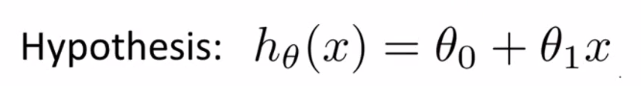里面有θ0和θ1两个参数，参数的改变将会导致假设函数的变化，比如：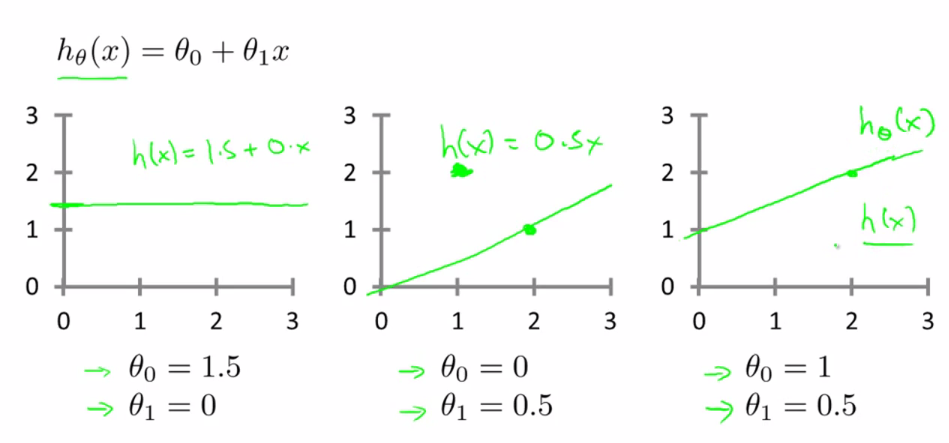现实的例子中，数据会以很多点的形式给我们，我们想要解决回归问题，就需要将这些点拟合成一条直线，找到最优的θ0和θ1来使这条直线更能代表所有数据。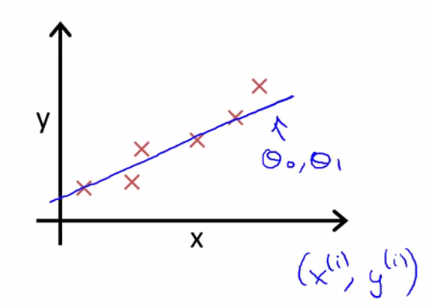而如何找到最优解呢，这就需要使用代价函数来求解了，以平方误差代价函数为例。
从最简单的单一参数来看，假设函数为：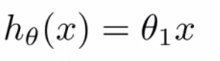平方误差代价函数的主要思想就是将实际数据给出的值与我们拟合出的线的对应值做差，这样就能求出我们拟合出的直线与实际的差距了。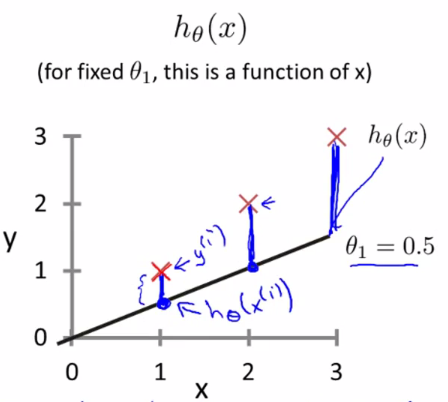而在前面乘以1/2，是因为后面求导会有2，为了简便计算。这样，就产生了代价函数：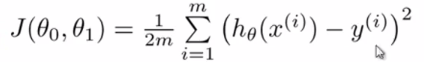而最优解即为代价函数的最小值，根据以上公式多次计算可得到
代价函数的图像：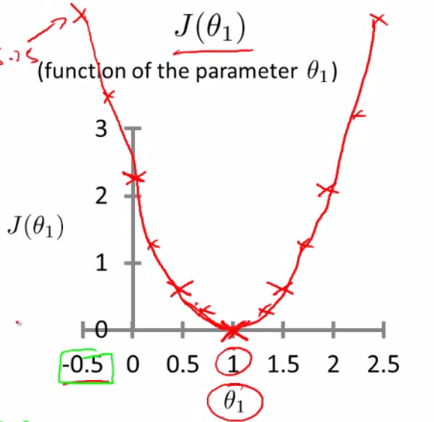可以看到该代价函数的确有最小值，这里恰好是横坐标为1的时候。
如果更多参数的话，就会更为复杂，两个参数的时候就已经是三维图像了：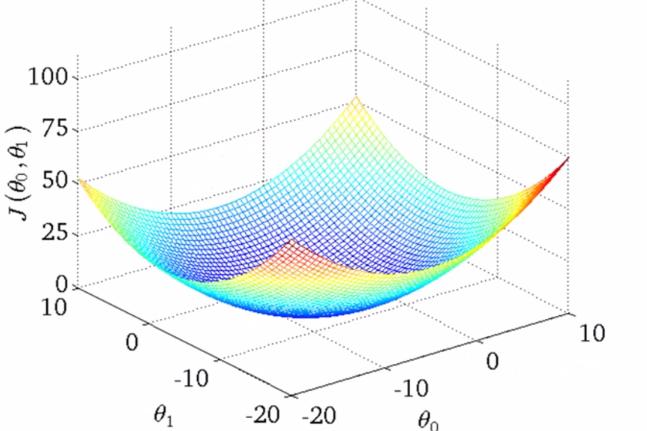高度即为代价函数的值，可以看到它仍然有着最小值的，而到达更多的参数的时候就无法像这样可视化了，但是原理都是相似的。
因此，对于回归问题，我们就可以归结为得到代价函数的最小值：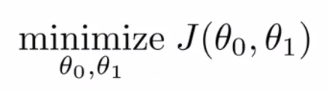这是我在学习ng的机器学习课程的基础上，经过自己的一些思考，写下学习笔记，重点是对于一些细节的思考和逻辑的理清。
以上很多都是个人见解，如果有不对的地方还请大家指点。


展开全文机器学习 线性回归
• 二次代价函数的局限性 首先来说一下二次代价函数的局限性，看下面这张图： 假设现在使用的激活函数是sigmoid，并且代价函数是二次代价函数，我们收敛得目标是1，那么A点的梯度比较大，B点由于离目标比较近梯度小，...
二次代价函数的局限性
首先来说一下二次代价函数的局限性，看下面这张图：假设现在使用的激活函数是sigmoid，并且代价函数是二次代价函数，我们收敛得目标是1，那么A点的梯度比较大，B点由于离目标比较近梯度小，所以是合理的；但是如果我们的收敛目标是0，那么A点离目标比较近，所以梯度应该要小于B点，但是图中明明是A点的梯度大于B点，这是不合理的。
交叉熵
正式由于二次大家函数的这个局限性，我们需要引入交叉熵。看下面这张图：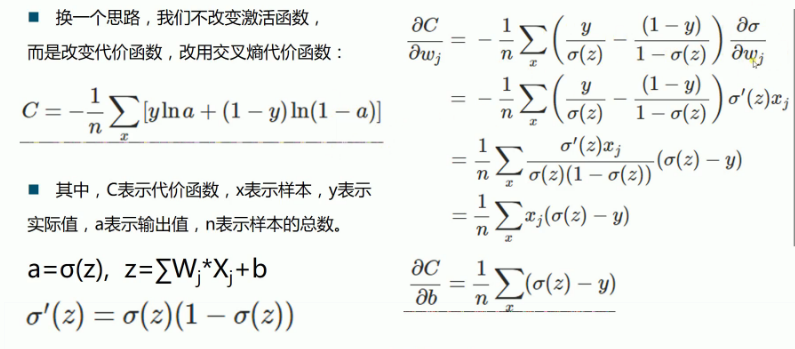上面这张图中，$C=-\frac{1}{2}\sum[ylna+(1-y)ln(1-a)]$代表的是交叉熵代价函数，对w和b进行求导后得到下面的式子：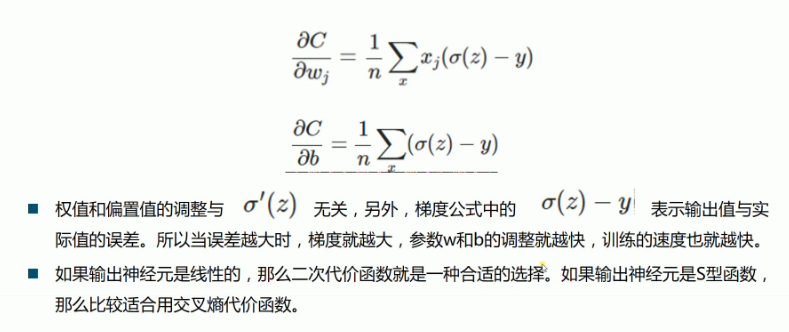现在我们发现w和b的调整已经跟 激活函数的导数（注意是导数哦） 无关了，而是跟输出值和真实值的误差有关，他们的误差越大，梯度也就越大，w和b的收敛速度越快。
总结
虽然二次代价函数有自身的局限性，但是我们也不能因此而丢弃它不用，因为在线性回归问题中它依然用后很大的价值，因此要根据实际需要选择代价函数。底下是一个小结，大家可以在实际中根据需要进行选择：

二次代价函数：输出神经元是线性的就选用该代价函数
交叉熵代价函数：输出神经元是S型的就选用该代价函数，例如sigmoid激活函数中（tf.nn.sigmoid_cross_entropy_with_logits()）
对数似然代价函数：输出神经元是softmax的时候选用（tf.nn.softmax_cross_entropy_with_logits()）

结束线/
欢迎大家加入Q群讨论：463255841
结束线/


展开全文• ## 代价函数总结

千次阅读 2017-09-22 16:06:18
代价函数是学习模型优化时的目标函数或者准则，通过最小化代价函数来优化模型。到目前为止，接触了一些机器学习算法，但是他们使用的代价函数不一定是一样的，由于，在现实的使用中，通常代价函数都需要自己来确定，...
代价函数是学习模型优化时的目标函数或者准则，通过最小化代价函数来优化模型。到目前为止，接触了一些机器学习算法，但是他们使用的代价函数不一定是一样的，由于，在现实的使用中，通常代价函数都需要自己来确定，所以，这里总结一下，代价函数都有哪些形式，尽量揣测一下，这样使用的原因。

1. 均方差代价函数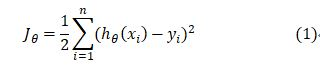这个是Andrew ng的机器学习课程里面看到的损失函数，在线性回归模型里面提出来的。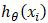表示模型所预测（假设）的输出，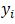是真实的输出，即label。
个人猜测，均方差应该是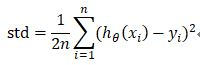由于对给定的数据集来说，n是确定的值，因此，可以等同于式(1)。

这个形式的代价函数计算Jacobian矩阵如下：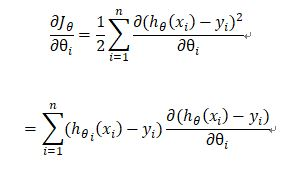2. 对数损失函数

对数似然作为代价函数是在RNN中看到的，公式如下：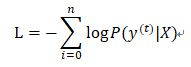表示真实目标在数据集中的条件概率的负对数。其意义在于，在很多预测目标概率的模型中，将最大概率对应的类型作为输出类型，因此，真实目标的预测概率越高，分类越准确，学习的目标是真实目标的预测概率最大化。而概率是小于1的，其对数值小于0，且对数是单调递增的，因此，当负对数最小化，就等同于对数最大化，概率最大化。
逻辑回归中的代价函数实际上就是对数似然的特殊表示的方式：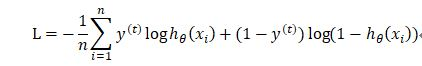二项逻辑回归的输入是预测目标为1的概率，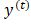的值为1或0.因此，目标为0的概率为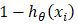，当真实的目标是1时，等式右边第二项为0，当真是目标为0时，等式右边第一项为0，因此，对于单个样本，L就是负对数似然。
同理，对于softmax回归的概率函数为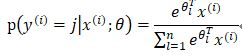未添加权重惩罚项的代价函数为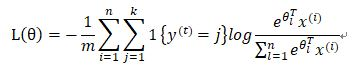3.交叉熵

交叉熵在神经网络中基本都用交叉熵作为代价函数。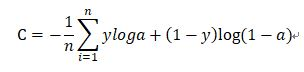这和逻辑回归的代价函数很像，y作为真实的目标（label），不一定是二值的，且a不是预测目标的概率，而是神经网络的输出，
它的原理还不是很明白，据说在神经网络中用交叉熵而不用均方差代价函数的原因是早期的神经元的激活函数是sigmoid函数，而此函数在大部分取值范围的导数都很小，这样使得参数的迭代很慢。
而交叉熵的产生过程网友是这样推导的：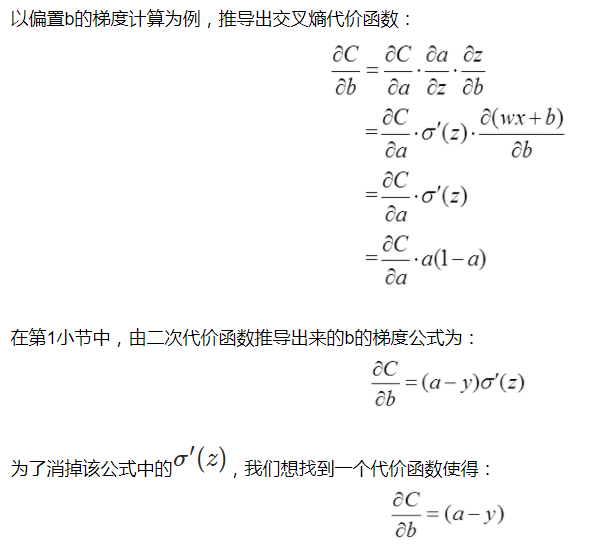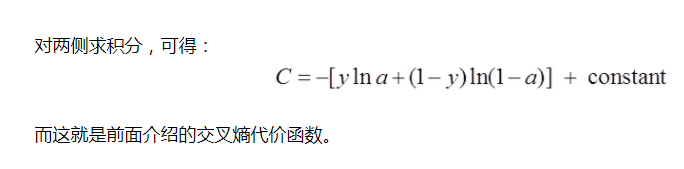展开全文• 参考知乎：https://www.zhihu.com/question/52398145基本概念：损失函数：计算的是一个样本的误差代价函数：是整个训练集上所有样本误差的平均目标函数：代价函数 + 正则化项原理：首先给出结论：损失函数和代价函数...
参考知乎：https://www.zhihu.com/question/52398145基本概念：损失函数：计算的是一个样本的误差代价函数：是整个训练集上所有样本误差的平均目标函数：代价函数 + 正则化项原理：首先给出结论：损失函数和代价函数是同一个东西，目标函数是一个与他们相关但更广的概念，对于目标函数来说在有约束条件下的最小化就是损失函数（loss function）。举个例子解释一下:（图片来自Andrew Ng Machine Learning公开课视频）&amp;lt;img src=&quot;https://pic3.zhimg.com/50/v2-3f4959cd70308df496ecc4568a0d982d_hd.jpg&quot; data-rawwidth=&quot;1772&quot; data-rawheight=&quot;524&quot; class=&quot;origin_image zh-lightbox-thumb&quot; width=&quot;1772&quot; data-original=&quot;https://pic3.zhimg.com/v2-3f4959cd70308df496ecc4568a0d982d_r.jpg&quot;&amp;gt;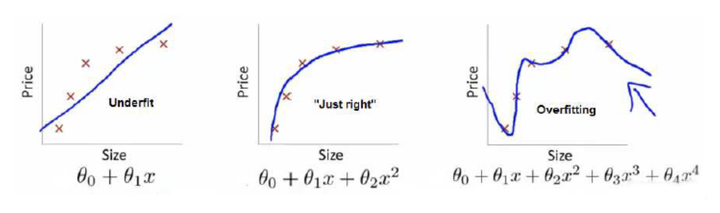上面三个图的函数依次为,,。我们是想用这三个函数分别来拟合Price，Price的真实值记为。我们给定，这三个函数都会输出一个,这个输出的与真实值可能是相同的，也可能是不同的，为了表示我们拟合的好坏，我们就用一个函数来度量拟合的程度，比如：，这个函数就称为损失函数(loss function)，或者叫代价函数(cost function)。损失函数越小，就代表模型拟合的越好。那是不是我们的目标就只是让loss function越小越好呢？还不是。这个时候还有一个概念叫风险函数(risk function)。风险函数是损失函数的期望，这是由于我们输入输出的遵循一个联合分布，但是这个联合分布是未知的，所以无法计算。但是我们是有历史数据的，就是我们的训练集，关于训练集的平均损失称作经验风险(empirical risk)，即，所以我们的目标就是最小化，称为经验风险最小化。到这里完了吗？还没有。如果到这一步就完了的话，那我们看上面的图，那肯定是最右面的的经验风险函数最小了，因为它对历史的数据拟合的最好嘛。但是我们从图上来看肯定不是最好的，因为它过度学习历史数据，导致它在真正预测时效果会很不好，这种情况称为过拟合(over-fitting)。为什么会造成这种结果？大白话说就是它的函数太复杂了，都有四次方了，这就引出了下面的概念，我们不仅要让经验风险最小化，还要让结构风险最小化。这个时候就定义了一个函数，这个函数专门用来度量模型的复杂度，在机器学习中也叫正则化(regularization)。常用的有,范数。到这一步我们就可以说我们最终的优化函数是：，即最优化经验风险和结构风险，而这个函数就被称为目标函数。结合上面的例子来分析：最左面的结构风险最小（模型结构最简单），但是经验风险最大（对历史数据拟合的最差）；最右面的经验风险最小（对历史数据拟合的最好），但是结构风险最大（模型结构最复杂）;而达到了二者的良好平衡，最适合用来预测未知数据集。以上的理解基于Coursera上Andrew Ng的公开课和李航的《统计学习方法》,如有理解错误，欢迎大家指正。前言交叉熵损失函数交叉熵损失函数的求导前言说明：本文只讨论Logistic回归的交叉熵，对Softmax回归的交叉熵类似。 首先，我们二话不说，先放出交叉熵的公式： J(θ)=−1m∑i=1my(i)log(hθ(x(i)))+(1−y(i))log(1−hθ(x(i))),J(θ)=−1m∑i=1my(i)log⁡(hθ(x(i)))+(1−y(i))log⁡(1−hθ(x(i))),以及J(θ)对J(θ)对参数θθ的偏导数（用于诸如梯度下降法等优化算法的参数更新），如下： ∂∂θjJ(θ)=1m∑i=1m(hθ(x(i))−y(i))x(i)j∂∂θjJ(θ)=1m∑i=1m(hθ(x(i))−y(i))xj(i)但是在大多论文或数教程中，也就是直接给出了上面两个公式，而未给出推导过程，而且这一过程并不是一两步就可以得到的，这就给初学者造成了一定的困惑，所以我特意在此详细介绍了它的推导过程，跟大家分享。因水平有限，如有错误，欢迎指正。交叉熵损失函数我们一共有m组已知样本，(x(i),y(i))(x(i),y(i))表示第 ii 组数据及其对应的类别标记。其中x(i)=(1,x(i)1,x(i)2,...,x(i)p)Tx(i)=(1,x1(i),x2(i),...,xp(i))T为p+1维向量（考虑偏置项），y(i)y(i)则为表示类别的一个数：logistic回归（是非问题）中，y(i)y(i)取0或者1；softmax回归（多分类问题）中，y(i)y(i)取1,2…k中的一个表示类别标号的一个数（假设共有k类）。这里，只讨论logistic回归，输入样本数据x(i)=(1,x(i)1,x(i)2,...,x(i)p)Tx(i)=(1,x1(i),x2(i),...,xp(i))T，模型的参数为θ=(θ0,θ1,θ2,...,θp)Tθ=(θ0,θ1,θ2,...,θp)T,因此有 θTx(i):=θ0+θ1x(i)1+⋯+θpx(i)p.θTx(i):=θ0+θ1x1(i)+⋯+θpxp(i).假设函数（hypothesis function）定义为： hθ(x(i))=11+e−θTx(i)hθ(x(i))=11+e−θTx(i). 因为Logistic回归问题就是0/1的二分类问题，可以有 P(y^(i)=1|x(i);θ)=hθ(x(i))P(y^(i)=1|x(i);θ)=hθ(x(i))P(y^(i)=0|x(i);θ)=1−hθ(x(i))P(y^(i)=0|x(i);θ)=1−hθ(x(i))现在，我们不考虑“熵”的概念，根据下面的说明，从简单直观角度理解，就可以得到我们想要的损失函数：我们将概率取对数，其单调性不变，有logP(y^(i)=1|x(i);θ)=loghθ(x(i))=log11+e−θTx(i),log⁡P(y^(i)=1|x(i);θ)=log⁡hθ(x(i))=log⁡11+e−θTx(i),logP(y^(i)=0|x(i);θ)=log(1−hθ(x(i)))=loge−θTx(i)1+e−θTx(i).log⁡P(y^(i)=0|x(i);θ)=log⁡(1−hθ(x(i)))=log⁡e−θTx(i)1+e−θTx(i).那么对于第ii组样本，假设函数表征正确的组合对数概率为： I{y(i)=1}logP(y^(i)=1|x(i);θ)+I{y(i)=0}logP(y^(i)=0|x(i);θ)=y(i)logP(y^(i)=1|x(i);θ)+(1−y(i))logP(y^(i)=0|x(i);θ)=y(i)log(hθ(x(i)))+(1−y(i))log(1−hθ(x(i)))I{y(i)=1}log⁡P(y^(i)=1|x(i);θ)+I{y(i)=0}log⁡P(y^(i)=0|x(i);θ)=y(i)log⁡P(y^(i)=1|x(i);θ)+(1−y(i))log⁡P(y^(i)=0|x(i);θ)=y(i)log⁡(hθ(x(i)))+(1−y(i))log⁡(1−hθ(x(i)))其中，I{y(i)=1}I{y(i)=1}和I{y(i)=0}I{y(i)=0}为示性函数（indicative function），简单理解为{ }内条件成立时，取1，否则取0，这里不赘言。 那么对于一共mm组样本，我们就可以得到模型对于整体训练样本的表现能力： ∑i=1my(i)log(hθ(x(i)))+(1−y(i))log(1−hθ(x(i)))∑i=1my(i)log⁡(hθ(x(i)))+(1−y(i))log⁡(1−hθ(x(i)))由以上表征正确的概率含义可知，我们希望其值越大，模型对数据的表达能力越好。而我们在参数更新或衡量模型优劣时是需要一个能充分反映模型表现误差的损失函数（Loss function）或者代价函数（Cost function）的，而且我们希望损失函数越小越好。由这两个矛盾，那么我们不妨领代价函数为上述组合对数概率的相反数： J(θ)=−1m∑i=1my(i)log(hθ(x(i)))+(1−y(i))log(1−hθ(x(i)))J(θ)=−1m∑i=1my(i)log⁡(hθ(x(i)))+(1−y(i))log⁡(1−hθ(x(i)))上式即为大名鼎鼎的交叉熵损失函数。(说明：如果熟悉“信息熵“的概念E[−logpi]=−∑mi=1pilogpiE[−log⁡pi]=−∑i=1mpilog⁡pi，那么可以有助理解叉熵损失函数）交叉熵损失函数的求导这步需要用到一些简单的对数运算公式，这里先以编号形式给出，下面推导过程中使用特意说明时都会在该步骤下脚标标出相应的公式编号，以保证推导的连贯性。 ①  logab=loga−logb  log⁡ab=log⁡a−log⁡b ②  loga+logb=log(ab)  log⁡a+log⁡b=log⁡(ab) ③  a=logea  a=log⁡ea 另外，值得一提的是在这里涉及的求导均为矩阵、向量的导数（矩阵微商），这里有一篇教程总结得精简又全面，非常棒，推荐给需要的同学。 下面开始推导： 交叉熵损失函数为： J(θ)=−1m∑i=1my(i)log(hθ(x(i)))+(1−y(i))log(1−hθ(x(i)))J(θ)=−1m∑i=1my(i)log⁡(hθ(x(i)))+(1−y(i))log⁡(1−hθ(x(i)))其中， loghθ(x(i))=log11+e−θTx(i)=−log(1+e−θTx(i)) ,log(1−hθ(x(i)))=log(1−11+e−θTx(i))=log(e−θTx(i)1+e−θTx(i))=log(e−θTx(i))−log(1+e−θTx(i))=−θTx(i)−log(1+e−θTx(i))①③ .log⁡hθ(x(i))=log⁡11+e−θTx(i)=−log⁡(1+e−θTx(i)) ,log⁡(1−hθ(x(i)))=log⁡(1−11+e−θTx(i))=log⁡(e−θTx(i)1+e−θTx(i))=log⁡(e−θTx(i))−log⁡(1+e−θTx(i))=−θTx(i)−log⁡(1+e−θTx(i))①③ .由此，得到 J(θ)=−1m∑i=1m[−y(i)(log(1+e−θTx(i)))+(1−y(i))(−θTx(i)−log(1+e−θTx(i)))]=−1m∑i=1m[y(i)θTx(i)−θTx(i)−log(1+e−θTx(i))]=−1m∑i=1m[y(i)θTx(i)−logeθTx(i)−log(1+e−θTx(i))]③=−1m∑i=1m[y(i)θTx(i)−(logeθTx(i)+log(1+e−θTx(i)))]②=−1m∑i=1m[y(i)θTx(i)−log(1+eθTx(i))]J(θ)=−1m∑i=1m[−y(i)(log⁡(1+e−θTx(i)))+(1−y(i))(−θTx(i)−log⁡(1+e−θTx(i)))]=−1m∑i=1m[y(i)θTx(i)−θTx(i)−log⁡(1+e−θTx(i))]=−1m∑i=1m[y(i)θTx(i)−log⁡eθTx(i)−log⁡(1+e−θTx(i))]③=−1m∑i=1m[y(i)θTx(i)−(log⁡eθTx(i)+log⁡(1+e−θTx(i)))]②=−1m∑i=1m[y(i)θTx(i)−log⁡(1+eθTx(i))]这次再计算J(θ)J(θ)对第jj个参数分量θjθj求偏导: ∂∂θjJ(θ)=∂∂θj(1m∑i=1m[log(1+eθTx(i))−y(i)θTx(i)])=1m∑i=1m[∂∂θjlog(1+eθTx(i))−∂∂θj(y(i)θTx(i))]=1m∑i=1m⎛⎝x(i)jeθTx(i)1+eθTx(i)−y(i)x(i)j⎞⎠=1m∑i=1m(hθ(x(i))−y(i))x(i)j∂∂θjJ(θ)=∂∂θj(1m∑i=1m[log⁡(1+eθTx(i))−y(i)θTx(i)])=1m∑i=1m[∂∂θjlog⁡(1+eθTx(i))−∂∂θj(y(i)θTx(i))]=1m∑i=1m(xj(i)eθTx(i)1+eθTx(i)−y(i)xj(i))=1m∑i=1m(hθ(x(i))−y(i))xj(i)这就是交叉熵对参数的导数： ∂∂θjJ(θ)=1m∑i=1m(hθ(x(i))−y(i))x(i)j∂∂θjJ(θ)=1m∑i=1m(hθ(x(i))−y(i))xj(i)转载请注明出处：http://blog.csdn.net/jasonzzj/article/details/52017438
展开全文• 交叉熵代价函数（Cross-entropy cost function）是用来衡量人工神经网络（ANN）的预测值与实际值的一种方式。与二次代价函数相比，它能更有效地促进ANN的训练。在介绍交叉熵代价函数之前，本文先简要介绍二次代价...
• 一、损失函数、代价函数、目标函数定义 首先给出结论： 损失函数（Loss Function ）是定义在单个样本上的，算的是一个样本的误差。 代价函数（Cost Function ）是定义在整个训练集上的，是所有样本误差的平均，也...机器学习 损失函数 目标函数
• ## 3.1 代价函数

千次阅读 2018-01-15 21:01:50
3.1 代价函数（cost function）  代价函数有助于将最可能的线性函数与我们的数据相拟合。在线性回归中，我们有一个这样的数据集，m表示训练集样本数，而我们的假设函数，也就是我们用来进行预测的函数，是图中所示...机器学习 coursera
• 损失函数或者代价函数的目的是：衡量模型的预测能力的好坏。 损失函数(Loss function)：是定义在单个训练样本上的，也就是就算一个样本的误差，比如我们想要分类，就是预测的类别和实际类别的区别，是一个样本的哦...
• ## 交叉熵代价函数

万次阅读 多人点赞 2015-03-13 13:18:56
1.从方差代价函数说起代价函数经常用方差代价函数（即采用均方误差MSE），比如对于一个神经元（单输入单输出，sigmoid函数）,定义其代价函数为： 其中y是我们期望的输出，a为神经元的实际输出【 a=σ(z), where z=wx...深度学习
• 目标函数、损失函数、代价函数...代价函数对每个参数的偏导数就是梯度下降中提到的梯度，防止过拟合时添加的正则化项也是加在代价函数后面的。在学习相关算法的过程中，对代价函数的理解...
• 代价函数在监督学习的回归问题中，代价函数就是用于找到最优解的目的函数，反应了预测函数的准确性。代价函数的值越小，说明在回归问题的中，计算机程序对数据拟合的越好。也就是假设函数越正确。比如，对于这个假设...
• =================第一部分 代价函数====================代价函数=损失函数 在机器学习中的每一种算法中...一、什么代价函数假设有训练样本(x, y)，模型h，参数θ。h(θ) = θTx（θT表示θ的转置）。（1）概况...
• ## 深度学习基础之代价函数

千次阅读 多人点赞 2019-07-16 13:03:03
在机器学习和深度学习中，代价函数非常重要。所以十分有必要弄个清楚代价函数相关的概念和性质。本文介绍了什么代价函数，然后列举了常用的三种代价函数，并对其中的二次代价函数和交叉熵代价函数进行了比较。机器学习 深度学习
• 1、代价函数什么？  理解的代价函数就是用于找到最优解的目的函数，这也是代价函数的作用。 2、代价函数作用原理   对于回归问题，我们需要求出代价函数来求解最优解，常用的是平方误差代价函数。  比如，...
• 一：损失函数，代价函数，目标函数定义首先给出结论：损失函数（Loss Function ）是定义在单个样本上的，算的是一个样本的误差。代价函数（Cost Function ）是定义在整个训练集上的，是所有样本误差的平均，也就是...
• 目标函数，损失函数和代价函数 基本概念： 损失函数：计算的是一个样本的误差 代价函数：是整个训练集上所有样本误差的平均 目标函数：代价函数 + 正则化项 通常机器学习每一个算法中都会有一个目标函数，算法...
• 逻辑回归和SoftMax的理解:逻辑回归逻辑回归代价函数 这是逻辑回归的代价函数. 理解这个代价函数: 假设函数hθ代表的是预测y=1的概率(因为假设二值分布满足伯努利分布) - 例如:如果将x=1输入假设函数当中,...
• 代价函数2.1 为什么需要代价函数2.2 代价函数的形式3. 代价函数的定义 神经网络和深度学习系列笔记： 传送门 【前言】 这下函数是算法好坏的评价标准之一。 所讨论的事件是数据实例的估计值和真值之间的差异的一些...损失函数 目标函数
• 什么代价函数是这个呢？首先思考：什么是代价？ 简单理解代价就是预测值和实际值之间的差距，那对于多个样本来说，就是差距之和。如果我们直接使用 ，这个公式看起来就是表示假设值和实际值只差，再将每一个样本...
• 交叉熵损失函数 交叉熵损失函数的求导 前言 说明：本文只讨论Logistic回归的交叉熵，对Softmax回归的交叉熵类似。 首先，我们二话不说，先放出交叉熵的公式： J(θ)=−1m∑i=1my(i)log(hθ(x(i)))+(1−y(i))...logistic回归 逻辑回归
• 机器学习中的目标函数、损失函数、代价函数什么区别？ 首先给出结论：损失函数和代价函数是同一个东西， 目标函数是一个与他们相关但更广的概念，对于目标函数来说在有约束条件下的最小化就是损失函数（loss ...
• 损失函数和代价函数是同一个东西，目标函数是一个与他们相关但更广的概念，对于目标函数来说在有约束条件下的最小化就是损失函数（loss function）举个例子解释一下:上面三个图的函数依次为 , , 。我们是想用这三个...
• 关于代价函数前面的系数为什么选1/2将在后续博客中给予解释。事实上它也可以是其他数，但是这里是为了数学计算上的方便 2.2代价函数的作用 引入这三种函数就可以求解出假设模型中的参数θ，如果知道了参数，这个...损失函数 目标函数
• 一：损失函数，代价函数，目标函数定义首先给出结论：损失函数（Loss Function ）是定义在单个样本上的，算的是一个样本的误差。代价函数（Cost Function ）是定义在整个训练集上的，是所有样本误差的平均，也就是...
• ## 代价函数的意义

千次阅读 2019-03-21 18:59:07
我们来复习上一节的知识： 假设函数：hθ(x)=θ0+θ1xh_\theta(x)=\theta_0+\theta_1xhθ​(x)=θ0​+θ...代价函数：J(θ0,θ1)=12m∑i=1i=m(h(xi)−yi)2J(\theta_0,\theta_1)=\frac{1}{2m}\sum_{i=1}^{i=m}(h(x^i)......